Скачать презентацию CHAPTER 9 Stocks and Their Valuation n Features

• Количество слайдов: 30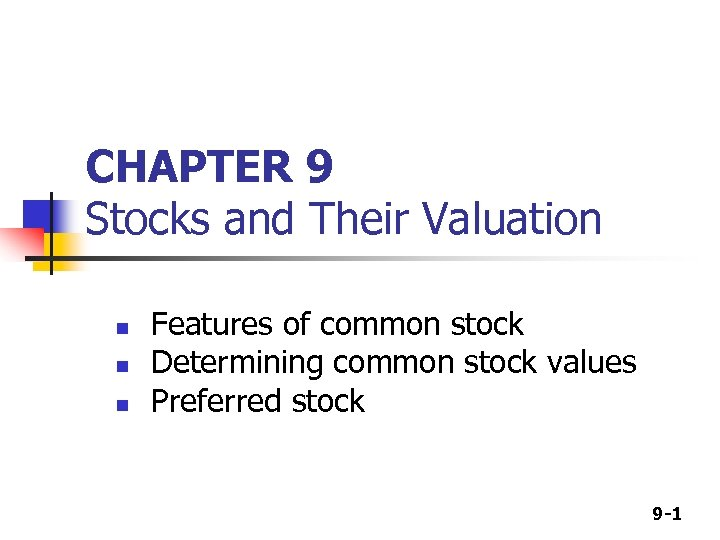CHAPTER 9 Stocks and Their Valuation n Features of common stock Determining common stock values Preferred stock 9 -1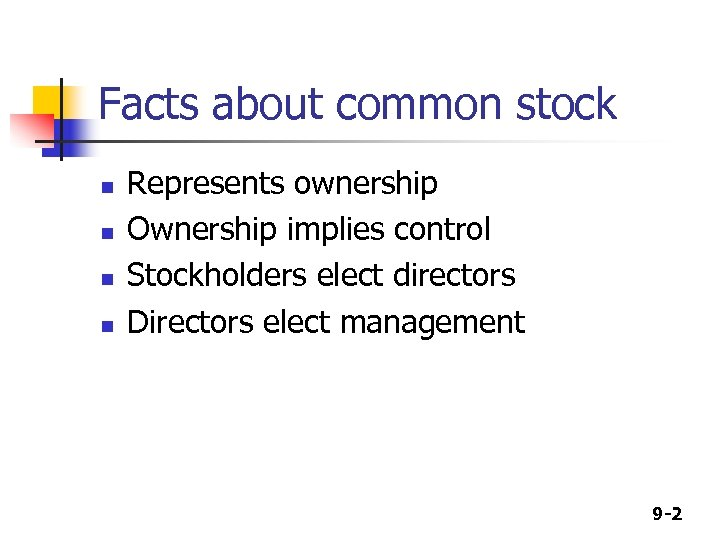Facts about common stock n n Represents ownership Ownership implies control Stockholders elect directors Directors elect management 9 -2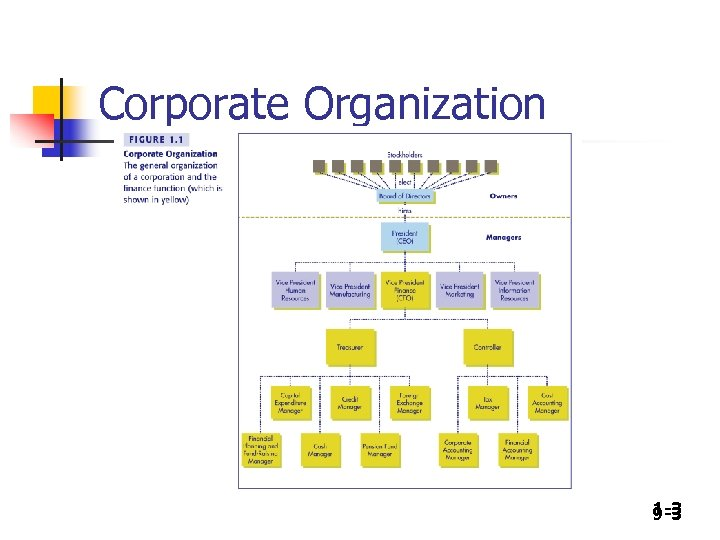Corporate Organization 1 -3 9 -3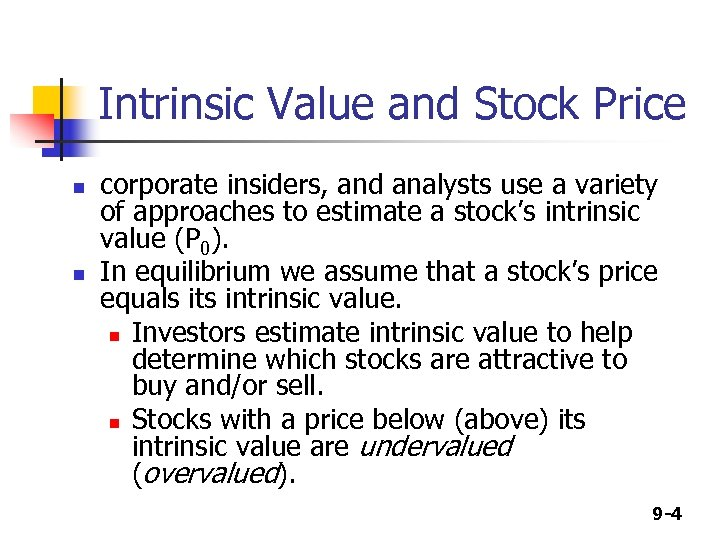Intrinsic Value and Stock Price n n corporate insiders, and analysts use a variety of approaches to estimate a stock’s intrinsic value (P 0). In equilibrium we assume that a stock’s price equals its intrinsic value. n Investors estimate intrinsic value to help determine which stocks are attractive to buy and/or sell. n Stocks with a price below (above) its intrinsic value are undervalued (overvalued). 9 -4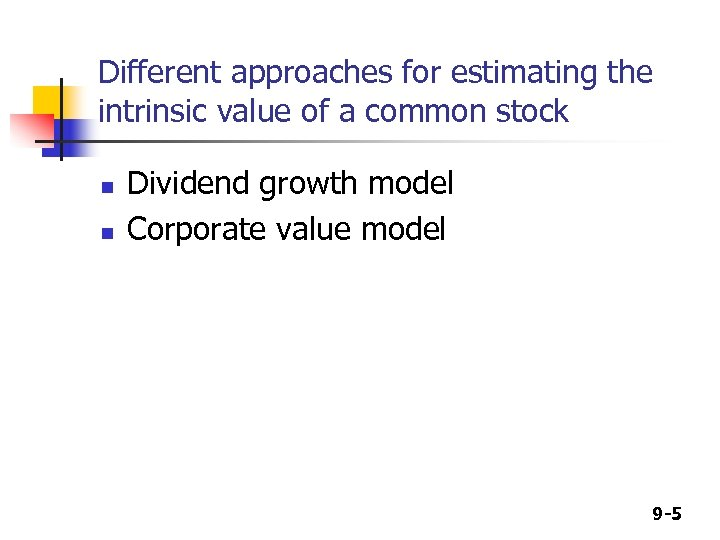Different approaches for estimating the intrinsic value of a common stock n n Dividend growth model Corporate value model 9 -5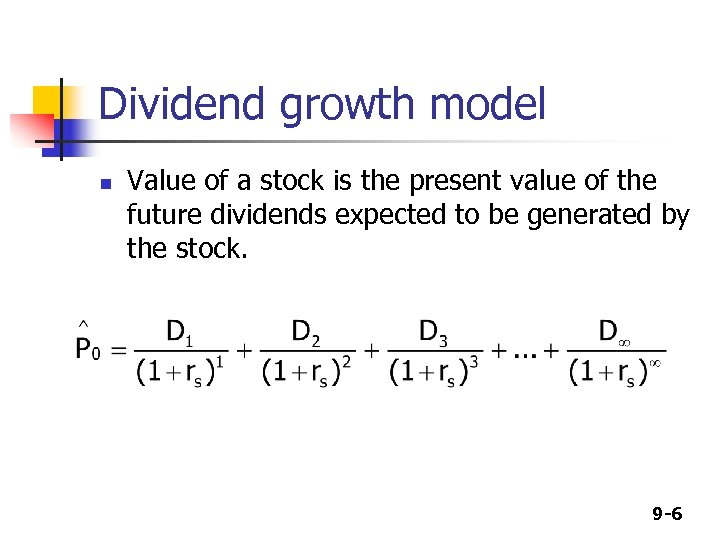Dividend growth model n Value of a stock is the present value of the future dividends expected to be generated by the stock. 9 -6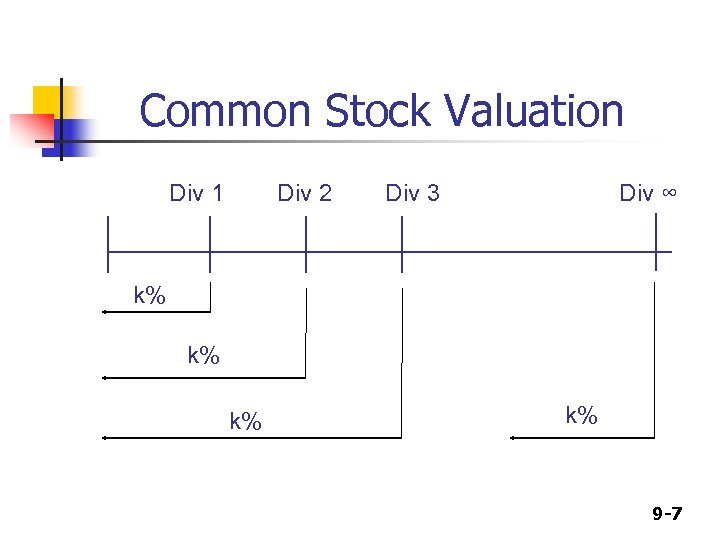Common Stock Valuation Div 1 Div 2 Div 3 Div ∞ k% k% 9 -7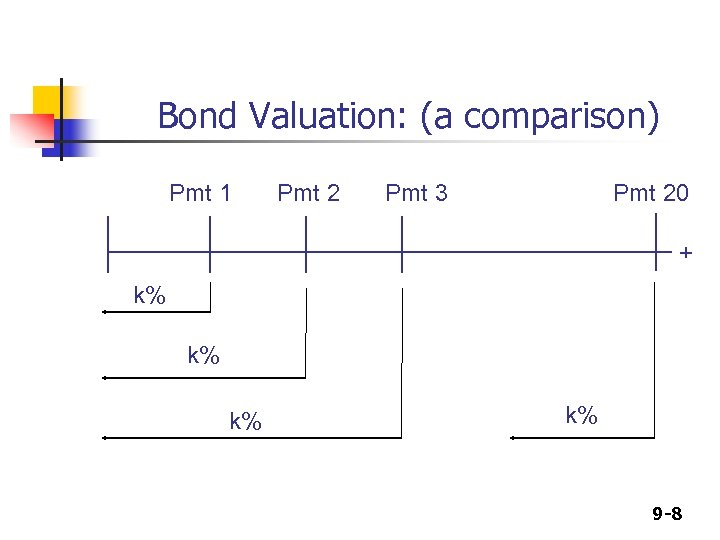Bond Valuation: (a comparison) Pmt 1 Pmt 2 Pmt 3 Pmt 20 + k% k% 9 -8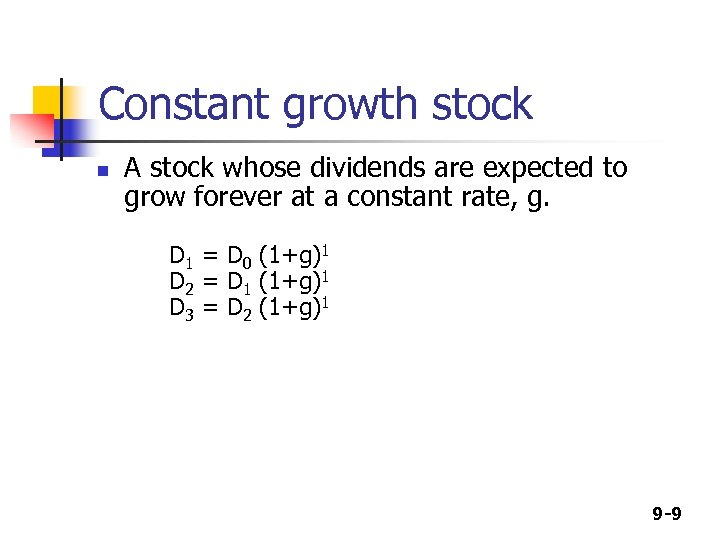Constant growth stock n A stock whose dividends are expected to grow forever at a constant rate, g. D 1 = D 0 (1+g)1 D 2 = D 1 (1+g)1 D 3 = D 2 (1+g)1 9 -9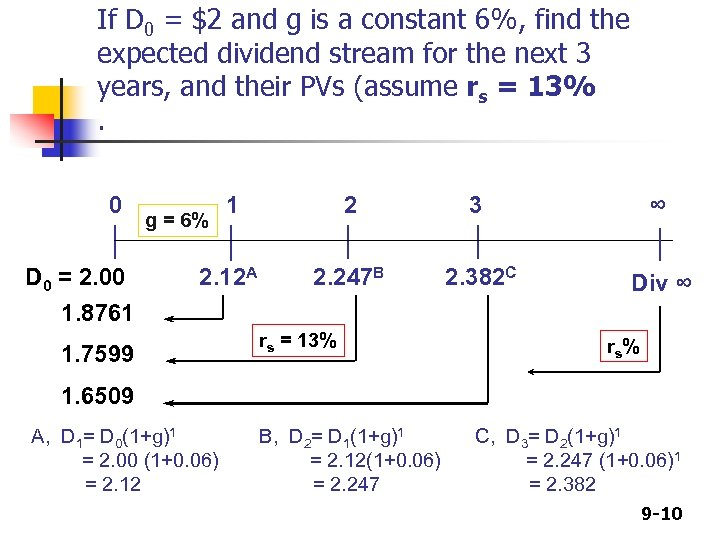If D 0 = \$2 and g is a constant 6%, find the expected dividend stream for the next 3 years, and their PVs (assume rs = 13%. 0 D 0 = 2. 00 1 2 3 ∞ 2. 12 A 2. 247 B 2. 382 C Div ∞ g = 6% 1. 8761 1. 7599 rs = 13% rs % 1. 6509 A, D 1= D 0(1+g)1 = 2. 00 (1+0. 06) = 2. 12 B, D 2= D 1(1+g)1 = 2. 12(1+0. 06) = 2. 247 C, D 3= D 2(1+g)1 = 2. 247 (1+0. 06)1 = 2. 382 9 -10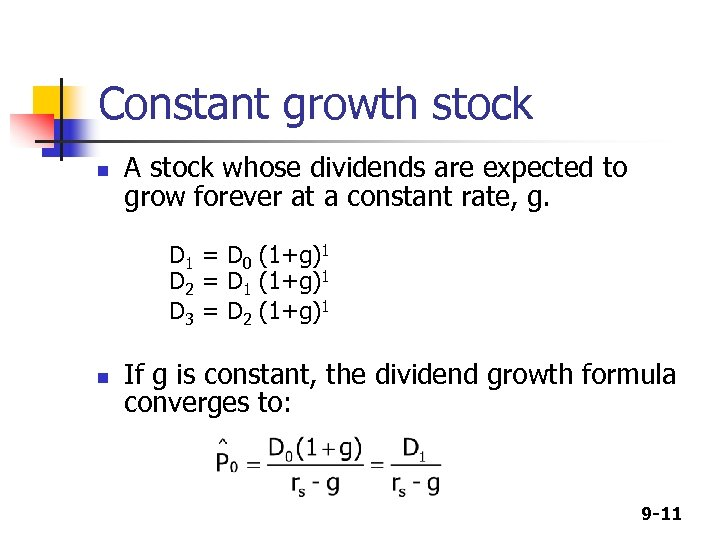Constant growth stock n A stock whose dividends are expected to grow forever at a constant rate, g. D 1 = D 0 (1+g)1 D 2 = D 1 (1+g)1 D 3 = D 2 (1+g)1 n If g is constant, the dividend growth formula converges to: 9 -11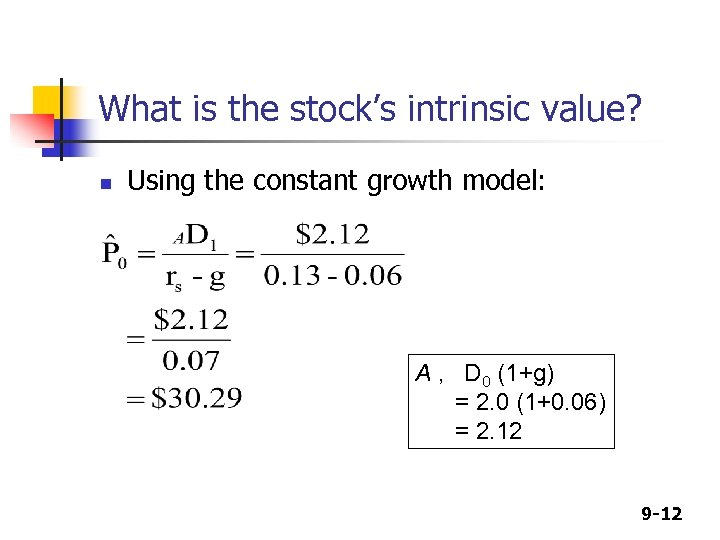What is the stock’s intrinsic value? n Using the constant growth model: A , D 0 (1+g) = 2. 0 (1+0. 06) = 2. 12 9 -12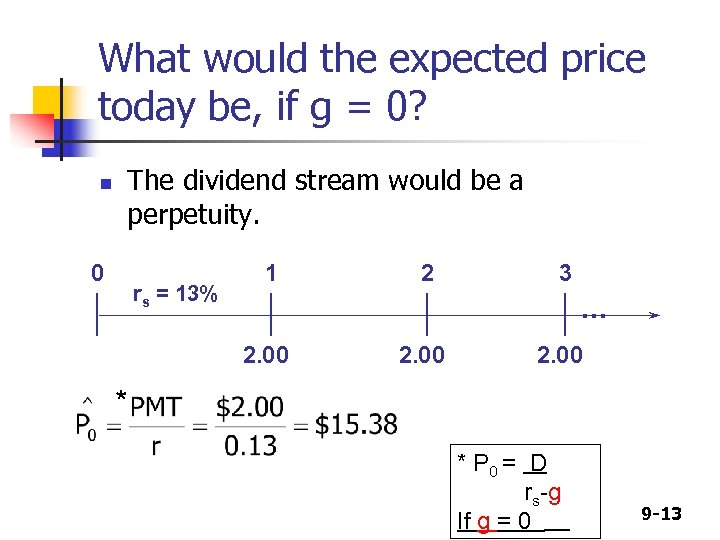What would the expected price today be, if g = 0? n The dividend stream would be a perpetuity. 0 rs = 13% 1 2 3 . . . 2. 00 * * P 0 = D rs-g If g = 0 9 -13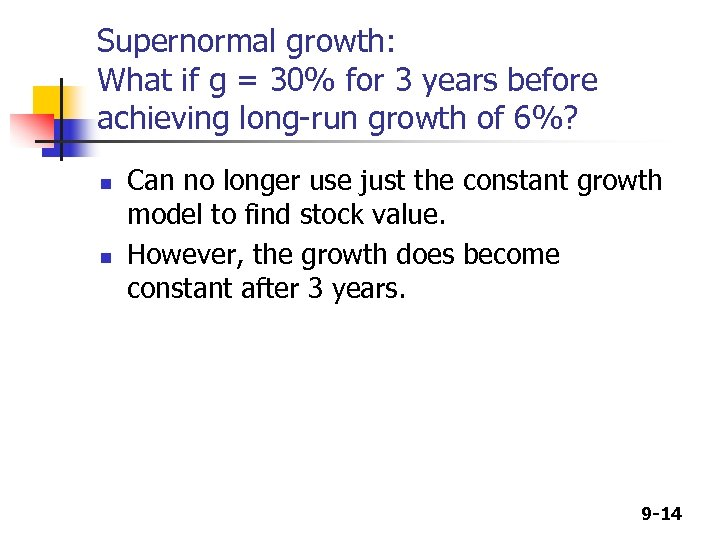Supernormal growth: What if g = 30% for 3 years before achieving long-run growth of 6%? n n Can no longer use just the constant growth model to find stock value. However, the growth does become constant after 3 years. 9 -14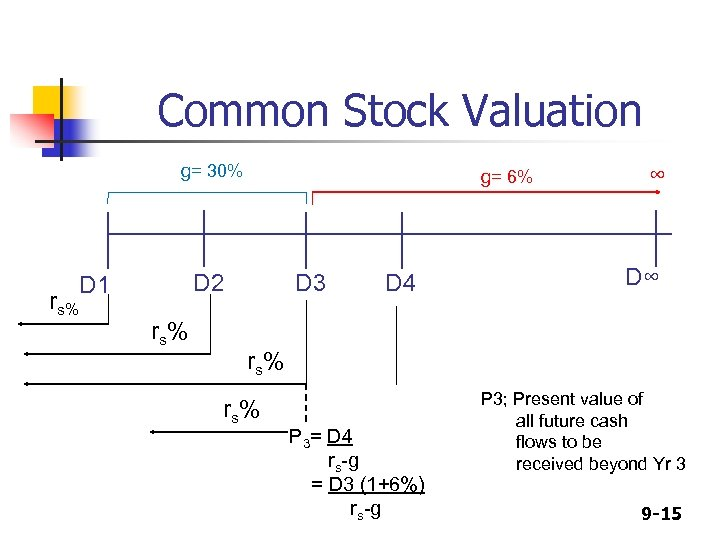Common Stock Valuation g= 30% rs% g= 6% D 2 D 1 rs% D 3 D 4 ∞ D∞ rs% P 3= D 4 rs-g = D 3 (1+6%) rs-g P 3; Present value of all future cash flows to be received beyond Yr 3 9 -15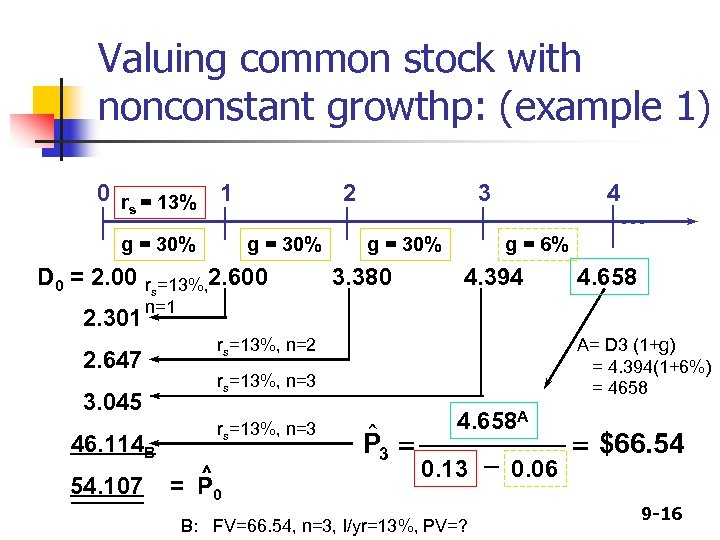Valuing common stock with nonconstant growthp: (example 1) 0 r = 13% 1 s g = 30% D 0 = 2. 00 2. 301 g = 30% rs=13%, 2. 600 n=1 3 g = 30% 3. 380 4 . . . g = 6% 4. 394 rs=13%, n=2 2. 647 rs=13%, n=3 46. 114 B ^ = P 0 4. 658 A= D 3 (1+g) = 4. 394(1+6%) = 4658 rs=13%, n=3 3. 045 54. 107 2 \$ P 3 = 4. 658 A 0. 13 - 0. 06 B: FV=66. 54, n=3, I/yr=13%, PV=? = \$66. 54 9 -16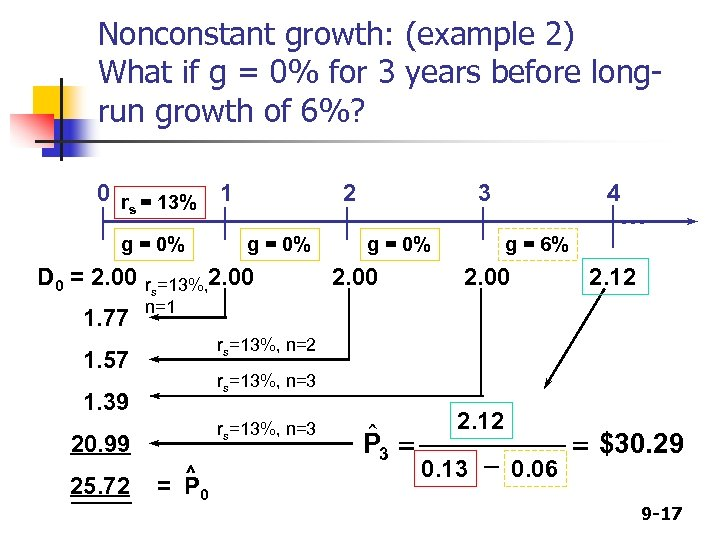Nonconstant growth: (example 2) What if g = 0% for 3 years before longrun growth of 6%? 0 r = 13% 1 s g = 0% D 0 = 2. 00 1. 77 g = 0% rs=13%, 2. 00 n=1 3 g = 0% 2. 00 4 . . . g = 6% 2. 00 2. 12 rs=13%, n=2 1. 57 rs=13%, n=3 1. 39 rs=13%, n=3 20. 99 25. 72 2 ^ = P 0 \$ P 3 = 2. 12 0. 13 - 0. 06 = \$30. 29 9 -17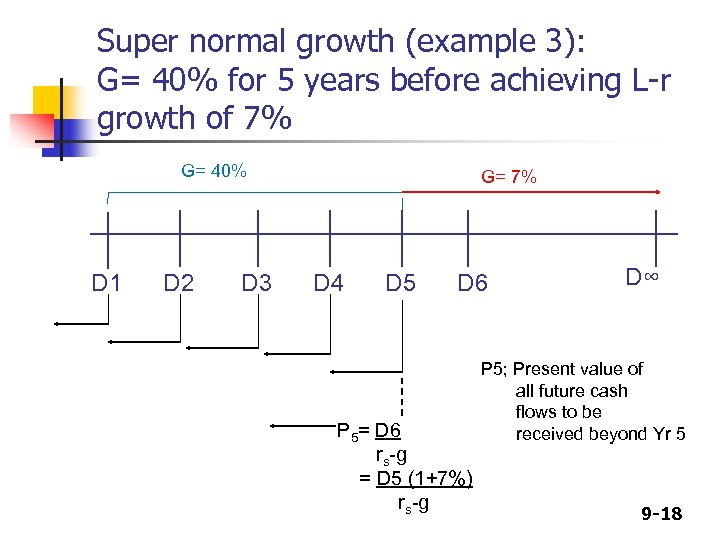Super normal growth (example 3): G= 40% for 5 years before achieving L-r growth of 7% G= 40% D 1 D 2 D 3 G= 7% D 4 D 5 D 6 P 5= D 6 rs-g = D 5 (1+7%) rs-g D∞ P 5; Present value of all future cash flows to be received beyond Yr 5 9 -18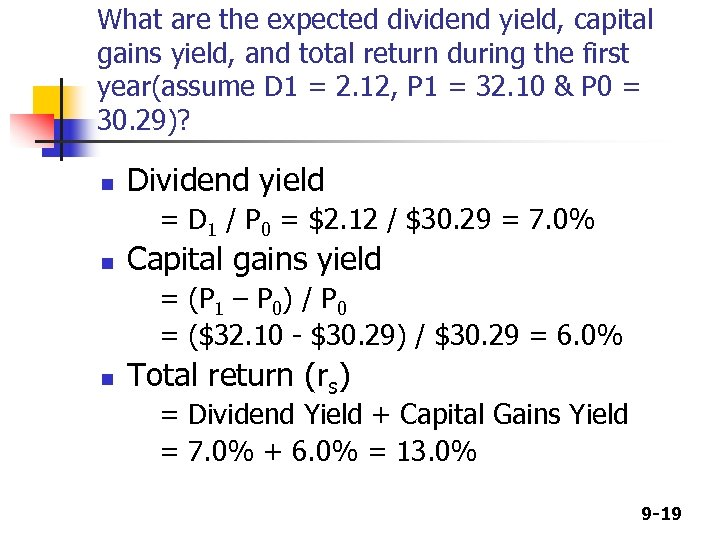What are the expected dividend yield, capital gains yield, and total return during the first year(assume D 1 = 2. 12, P 1 = 32. 10 & P 0 = 30. 29)? n Dividend yield = D 1 / P 0 = \$2. 12 / \$30. 29 = 7. 0% n Capital gains yield = (P 1 – P 0) / P 0 = (\$32. 10 - \$30. 29) / \$30. 29 = 6. 0% n Total return (rs) = Dividend Yield + Capital Gains Yield = 7. 0% + 6. 0% = 13. 0% 9 -19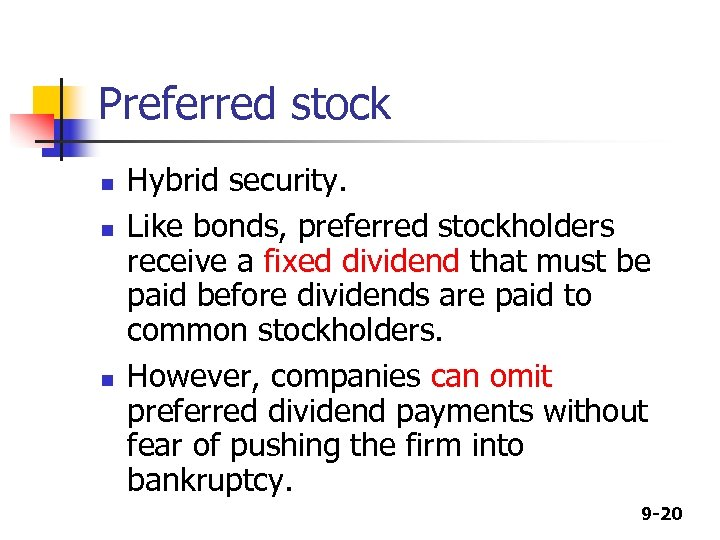Preferred stock n n n Hybrid security. Like bonds, preferred stockholders receive a fixed dividend that must be paid before dividends are paid to common stockholders. However, companies can omit preferred dividend payments without fear of pushing the firm into bankruptcy. 9 -20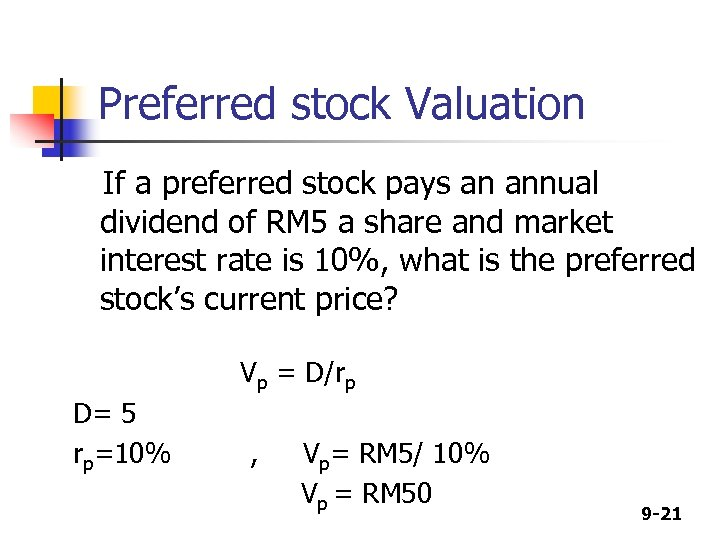Preferred stock Valuation If a preferred stock pays an annual dividend of RM 5 a share and market interest rate is 10%, what is the preferred stock’s current price? Vp = D/rp D= 5 rp=10% , Vp= RM 5/ 10% Vp = RM 50 9 -21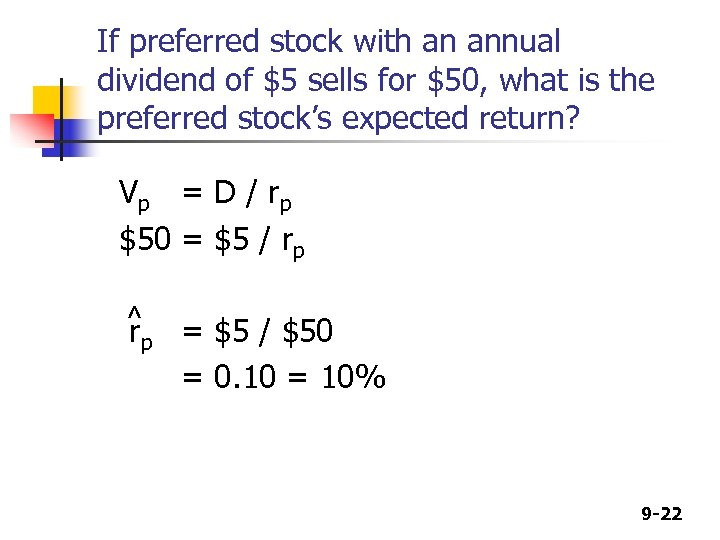If preferred stock with an annual dividend of \$5 sells for \$50, what is the preferred stock’s expected return? V p = D / rp \$50 = \$5 / rp ^ = \$5 / \$50 rp = 0. 10 = 10% 9 -22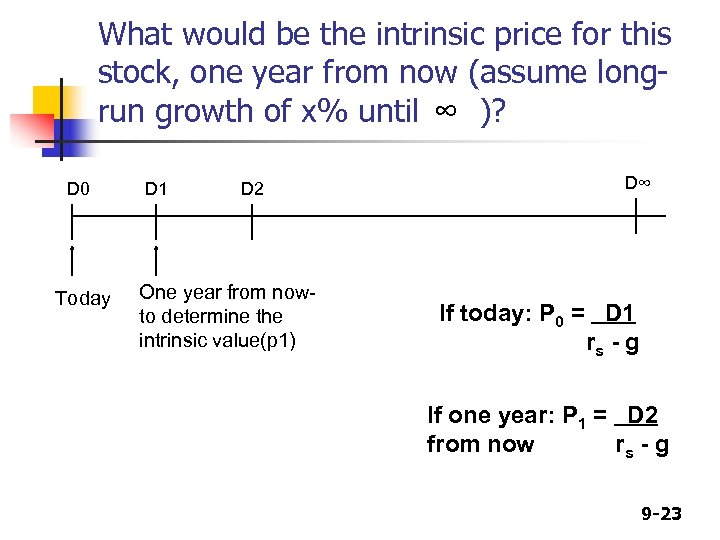What would be the intrinsic price for this stock, one year from now (assume longrun growth of x% until ∞ )? D 0 Today D 1 D 2 One year from nowto determine the intrinsic value(p 1) D∞ If today: P 0 = D 1 rs - g If one year: P 1 = D 2 from now rs - g 9 -23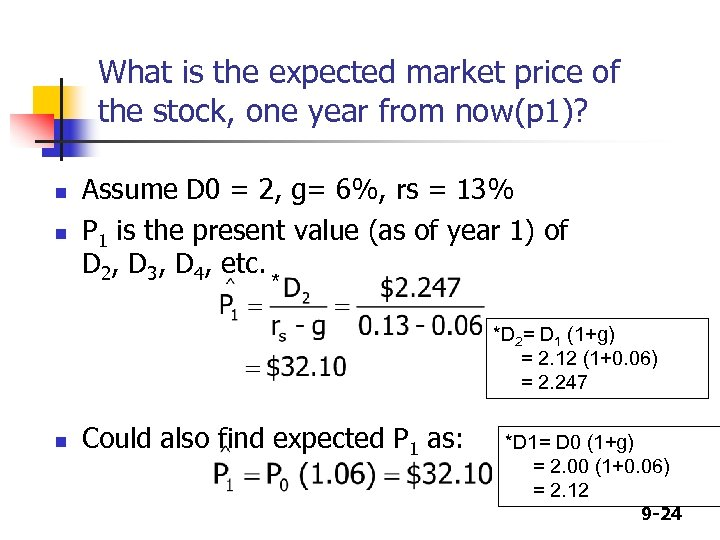What is the expected market price of the stock, one year from now(p 1)? n n Assume D 0 = 2, g= 6%, rs = 13% P 1 is the present value (as of year 1) of D 2, D 3, D 4, etc. * *D 2= D 1 (1+g) = 2. 12 (1+0. 06) = 2. 247 n Could also find expected P 1 as: *D 1= D 0 (1+g) = 2. 00 (1+0. 06) = 2. 12 9 -24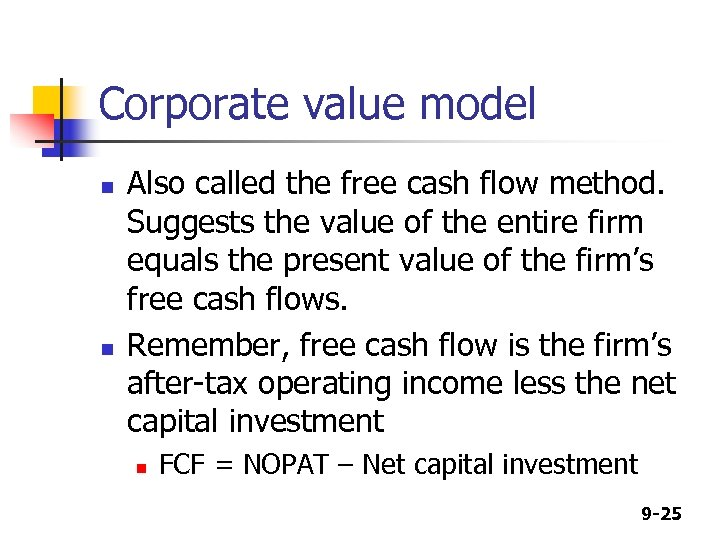Corporate value model n n Also called the free cash flow method. Suggests the value of the entire firm equals the present value of the firm’s free cash flows. Remember, free cash flow is the firm’s after-tax operating income less the net capital investment n FCF = NOPAT – Net capital investment 9 -25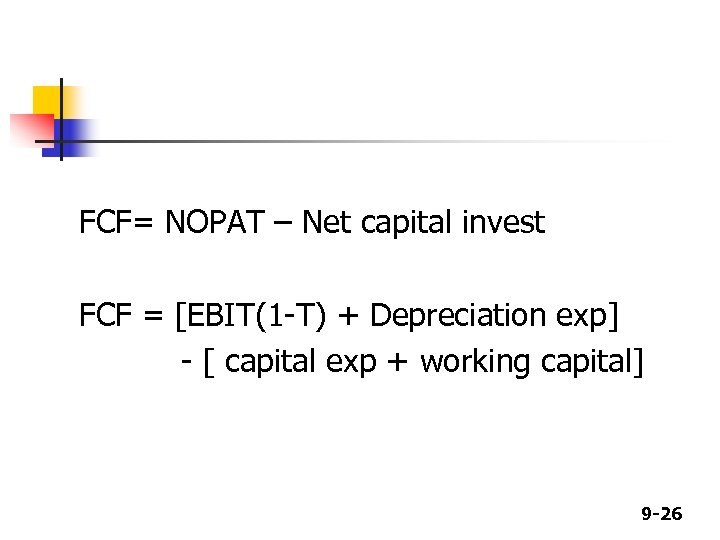FCF= NOPAT – Net capital invest FCF = [EBIT(1 -T) + Depreciation exp] - [ capital exp + working capital] 9 -26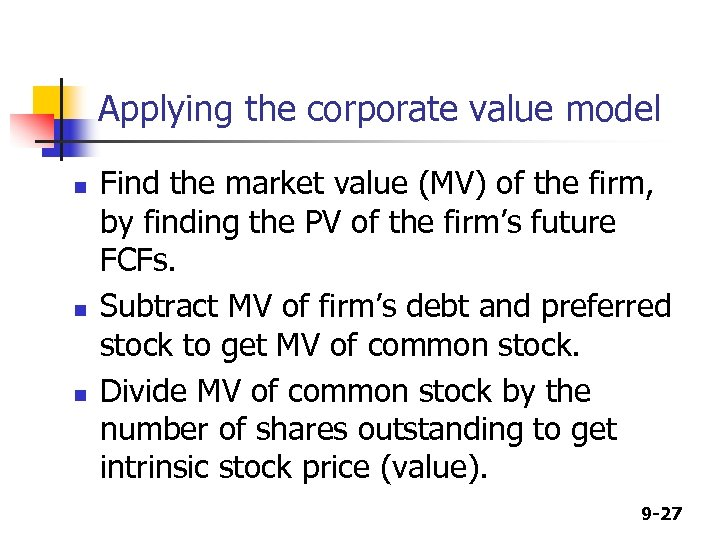Applying the corporate value model n n n Find the market value (MV) of the firm, by finding the PV of the firm’s future FCFs. Subtract MV of firm’s debt and preferred stock to get MV of common stock. Divide MV of common stock by the number of shares outstanding to get intrinsic stock price (value). 9 -27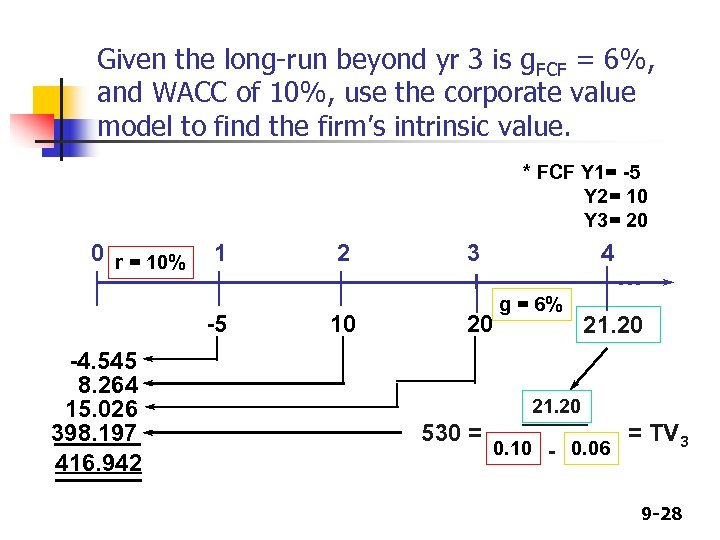Given the long-run beyond yr 3 is g. FCF = 6%, and WACC of 10%, use the corporate value model to find the firm’s intrinsic value. * FCF Y 1= -5 Y 2= 10 Y 3= 20 0 r = 10% 1 -5 -4. 545 8. 264 15. 026 398. 197 416. 942 2 10 3 4 20 g = 6% . . . 21. 20 530 = 0. 10 - 0. 06 = TV 3 9 -28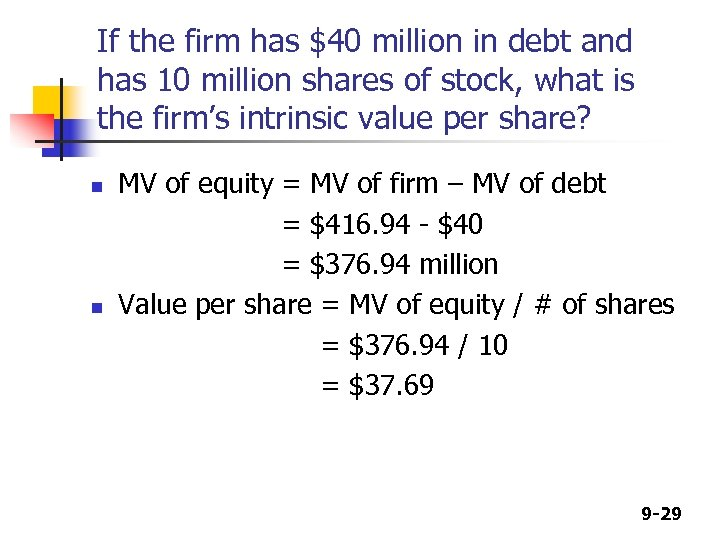If the firm has \$40 million in debt and has 10 million shares of stock, what is the firm’s intrinsic value per share? n n MV of equity = MV of firm – MV of debt = \$416. 94 - \$40 = \$376. 94 million Value per share = MV of equity / # of shares = \$376. 94 / 10 = \$37. 69 9 -29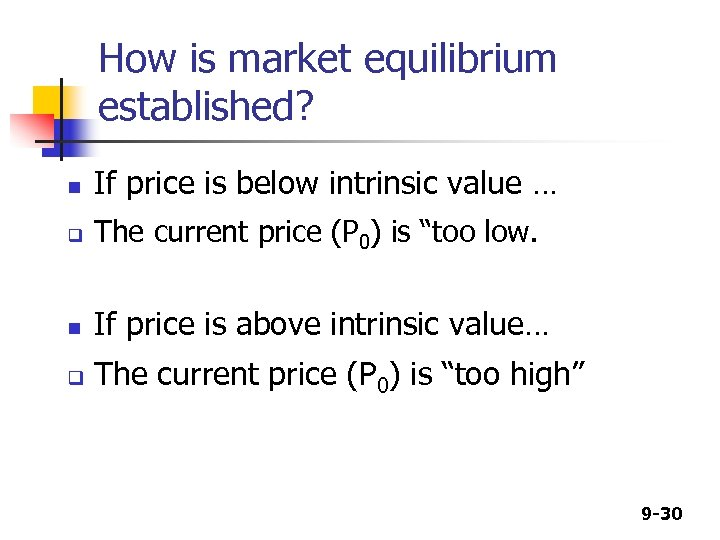How is market equilibrium established? n If price is below intrinsic value … q The current price (P 0) is “too low. n If price is above intrinsic value… q The current price (P 0) is “too high” 9 -30